## Introduction

Ok, I’m going to try to build some rough mathematical description of the game Jenga

You know Jenga, the game where you have a tower of little wooden blocks, and players take turns removing one block at a time from the tower, stack it on the top. The last player to stack a block without making the tower fall wins the game.

Right, well, might as well get down to it.

## Row Density

Ok, on any given row there are 3 blocks. If either of the side blocks are picked, then you can remove another block (i.e., there’s a 66% chance you’ll remove two blocks), and if you pick one from the centre, then you can not remover any other blocks from the stack (i.e., there’s a 33% chance you’ll remove only one block from a given row).

Formulating this, the average no of pieces you’ll take out of any given row is:This also means that, on average, it will take 5/3 turns to exhaust any row.

So, given R rows, you will on average take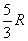turns to render the whole stack useless.

However, after each turn you will stack one block on top, so you will also have addedpieces on top of the stack, or have created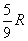new rows. With these rows, you will take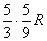turns to clear them and will generate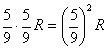extra rows, and these extra rows will generate, etc. etc.

So, in total, you will end up with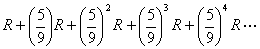Rows by the end (note that in reality that after a certain term the terms in the sequence become less than 1, and so the above series is an overestimation).

This is a geometric series, with a=R and r=5/9, and the sum is given as: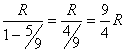Now, each of the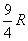rows take up roughly 5/3 turns, so the total number of turns it will take to finish up the tower is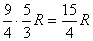turns.

Now, we know that the total number of blocks in the tower at any one time is constant, but the total number of moveable blocks in the tower reduces every turn, it goes down by one every turn, but goes up every three turns because of an extra row being added.

Thus, denoting the number of free blocks as a function of time: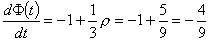This just means that every turn the no of moveable blocks decreases by an average of 4/9. (remember that the extra row being added does not create three extra moveable pieces, it creates, 5/3 extra moveable pieces on average)

So, given that the initial number of moveable blocks is, we can writeNow we can go on to some of the proper maths :)

## Probability Distribution

Now, taking any area of the tower, the chance that the person will pick a block from that area is proportional to the ration of the size of that area to the size of the whole tower, or, taking that area to be a specific row in it’s initial state:

P(Will be picked)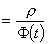Now, let D be the average number of blocks on a given row as a function of time.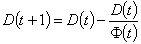or, simplifying:.

Now, expanding it a little, a pattern will emerge:But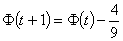soAnd so on…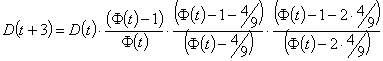And so, by induction: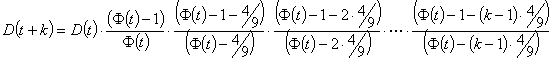Ok, all you need to know is that the gamma function has the property that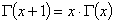for all.

Now, lets look at part of D(t+k), namely the lower half of the D coefficient: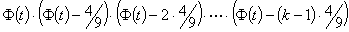Let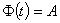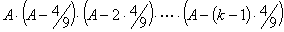Now, look at the following:Expanding this further: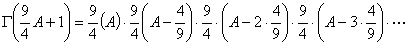But this sequence does not end. We have to cap it be dividing by another function that will cancel out all terms after.

So, let’s look at the following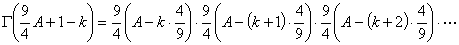Dividing one by the other, we getNow, we can substitute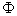for A and divide byto get the thing quotiented at the start of this section:and â€“ in addition, noting that the top half of the D coefficient from the previous section is of the same form with A as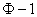.

## Back to the Distribution

So, we get the following functionAnd the two fractions cancel out givingWhich I think is pretty neat (coz I’ve never had a reason to use the Gamma function before, and coz gamma functions are neat :) )

Now, the rest is trivial:

Given an initial stack size of R, an additional row is created every three turns, so, rephrasing D as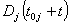where j is the row you’re looking for the number of pieces left to move, andis the turn on which the jth row was created.Now, the average number of blocks left to be moved on a row j can be approximated (realising that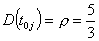) asAnd that’s as much as I’m going to do with it. You wouldn’t believe how many weird roads I had to go down to find the above solution, and I’m sure as hell not going to go actively looking for any more geometric sequences for quite some time (I was going mad yesterday trapped in a maze of them!).

Hope it made some sense, will clarify anything if you feel that anything’s badly explained.

1. Taylor wrote:

how can i turn this into a science fair project? I am doing something called math expo, where I have to do a science fair project, except it has to be math. I would like to do my project on this…

Sunday, January 13, 2013 at 9:19 pm | Permalink
2. Sure, that’d be awesome! Be sure to cite your sources, and double check everything to make sure I’m not talking bullshit :)

(also, please send me pictures if you do!)

Sunday, January 13, 2013 at 9:44 pm | Permalink

hey im going to be using this for my maths explorations, so i just wanted to confirm what conclusions did u draw from this?

Wednesday, October 30, 2013 at 6:45 am | Permalink
4. The calculation is an approximation of what the density of blocks during a game will tend to be. I didn’t draw any conclusions from this, but one can produce some nice graphs :).

Wednesday, October 30, 2013 at 1:12 pm | Permalink

Can u please explain this further for me ,i am having difficulties understanding some of the stuff. it would be really helpful if you could, it would be a great help for my assessment.

Tuesday, December 3, 2013 at 12:48 pm | Permalink

like can you please simplify the equations, this math seems to be of a much higher level. :)

Tuesday, December 3, 2013 at 12:50 pm | Permalink
7. If you have a particular question about a particular equation, I might be able to offer you a pointer, but I can’t just explain everything in simpler terms – I don’t have time for that.

Wednesday, December 4, 2013 at 2:04 am | Permalink
8. Patricia wrote:

We havent cover this level od probability of distribution but I would like to study this for my project.. Could you email me on how to graph the final equation? And what the variable on the final equation is? Thank you so much.. I’ll be sure to cite your work..

Thursday, January 23, 2014 at 10:57 pm | Permalink
9. Nupur wrote:

Hi! I’d like to use this for my math explorations and was wondering how to graph these equations? Could you please help me with that? thank you :)
ps- also, I didn’t fully understand what you were aiming to find in this experiment. Could you please correspond via email? thank you

Friday, March 21, 2014 at 1:02 pm | Permalink
10. If you have a particular question i can try answer, but this is too vague – I don’t have the time to try explain everything.

Saturday, March 22, 2014 at 12:43 pm | Permalink
11. Nupur wrote:

I meant, could you give me some examples of graphs that could be made with the data? Thank you :)

Sunday, March 23, 2014 at 8:33 am | Permalink
12. Nope, sorry.
Best,
S

Sunday, March 23, 2014 at 3:21 pm | Permalink

What are you trying to say. There will be no probability here as there are not defined number of trials and there are no rules in which if we take out the edge blocks then we can take out one more block

Monday, November 20, 2017 at 4:36 pm | Permalink